Скачать презентацию Chapter 11 Standard Costs and Variance Analysis

b83d5775ba49f07ed6dbd09b171f8054.ppt

• Количество слайдов: 20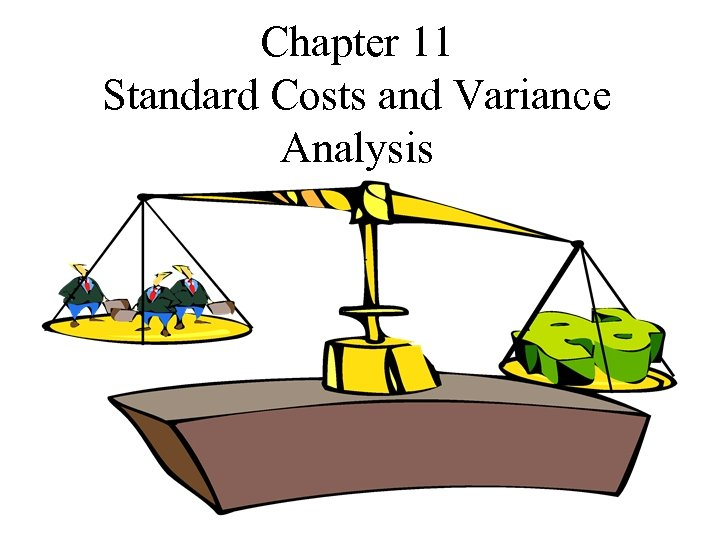Chapter 11 Standard Costs and Variance Analysis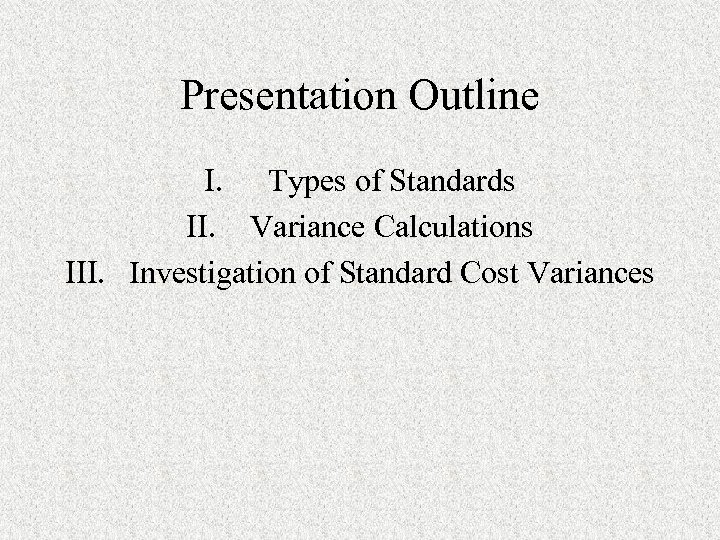Presentation Outline I. Types of Standards II. Variance Calculations III. Investigation of Standard Cost Variances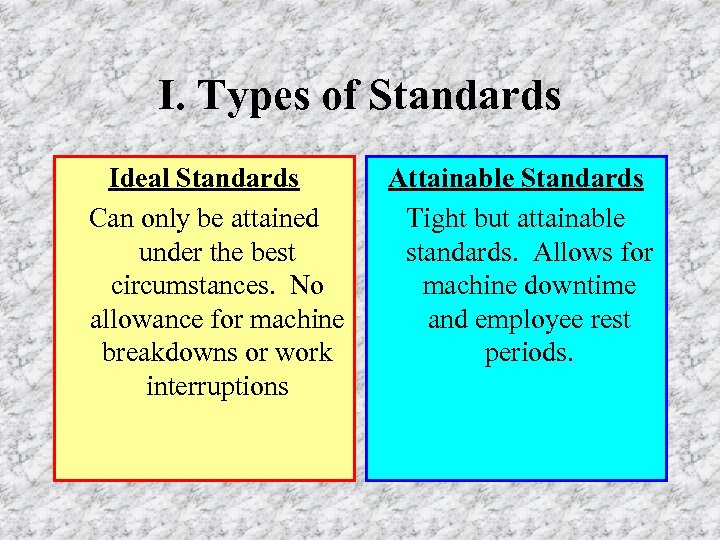I. Types of Standards Ideal Standards Can only be attained under the best circumstances. No allowance for machine breakdowns or work interruptions Attainable Standards Tight but attainable standards. Allows for machine downtime and employee rest periods.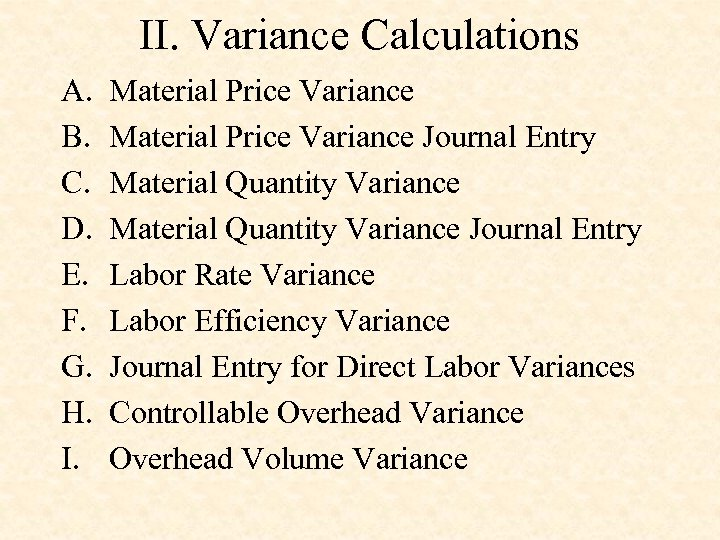II. Variance Calculations A. B. C. D. E. F. G. H. I. Material Price Variance Journal Entry Material Quantity Variance Journal Entry Labor Rate Variance Labor Efficiency Variance Journal Entry for Direct Labor Variances Controllable Overhead Variance Overhead Volume Variance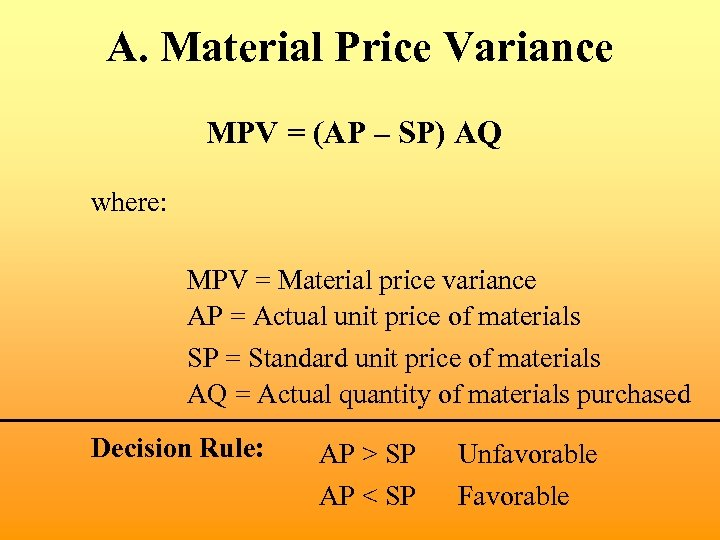A. Material Price Variance MPV = (AP – SP) AQ where: MPV = Material price variance AP = Actual unit price of materials SP = Standard unit price of materials AQ = Actual quantity of materials purchased Decision Rule: AP > SP AP < SP Unfavorable Favorable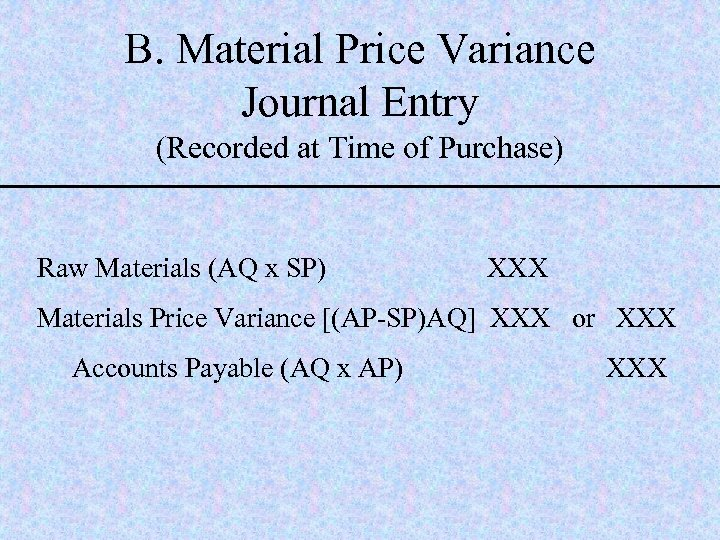B. Material Price Variance Journal Entry (Recorded at Time of Purchase) Raw Materials (AQ x SP) XXX Materials Price Variance [(AP-SP)AQ] XXX or XXX Accounts Payable (AQ x AP) XXX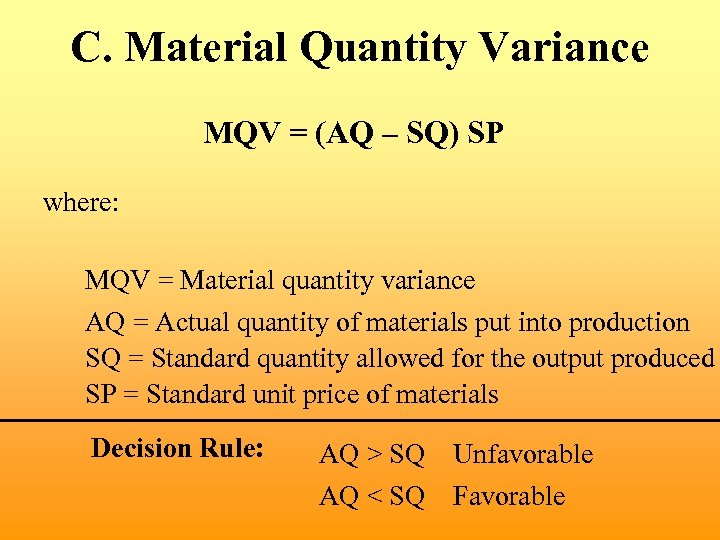C. Material Quantity Variance MQV = (AQ – SQ) SP where: MQV = Material quantity variance AQ = Actual quantity of materials put into production SQ = Standard quantity allowed for the output produced SP = Standard unit price of materials Decision Rule: AQ > SQ AQ < SQ Unfavorable Favorable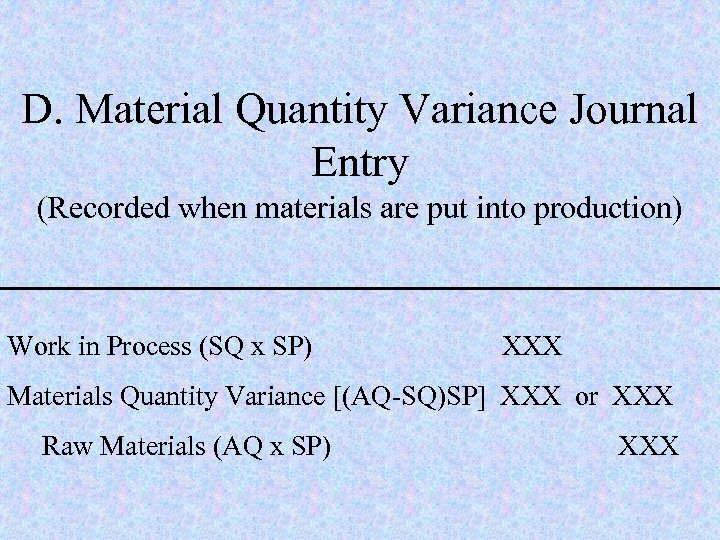D. Material Quantity Variance Journal Entry (Recorded when materials are put into production) Work in Process (SQ x SP) XXX Materials Quantity Variance [(AQ-SQ)SP] XXX or XXX Raw Materials (AQ x SP) XXX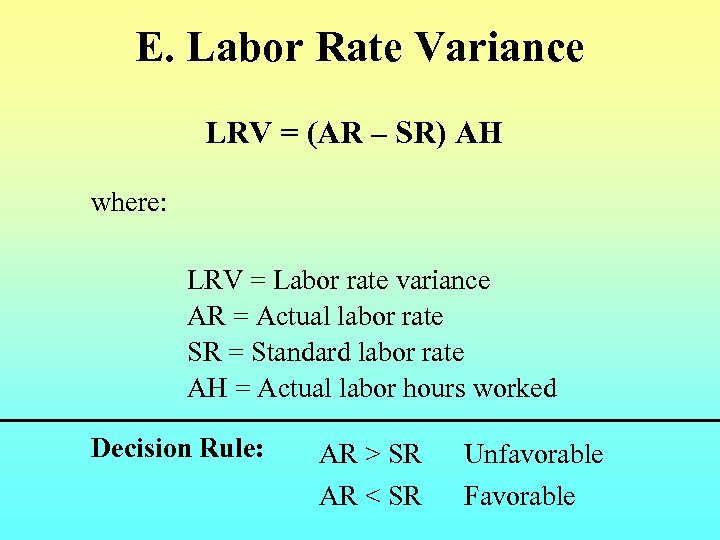E. Labor Rate Variance LRV = (AR – SR) AH where: LRV = Labor rate variance AR = Actual labor rate SR = Standard labor rate AH = Actual labor hours worked Decision Rule: AR > SR AR < SR Unfavorable Favorable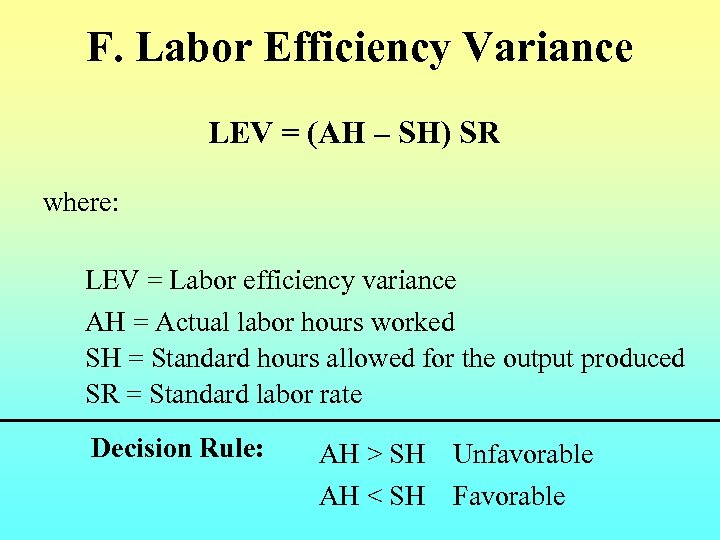F. Labor Efficiency Variance LEV = (AH – SH) SR where: LEV = Labor efficiency variance AH = Actual labor hours worked SH = Standard hours allowed for the output produced SR = Standard labor rate Decision Rule: AH > SH AH < SH Unfavorable Favorable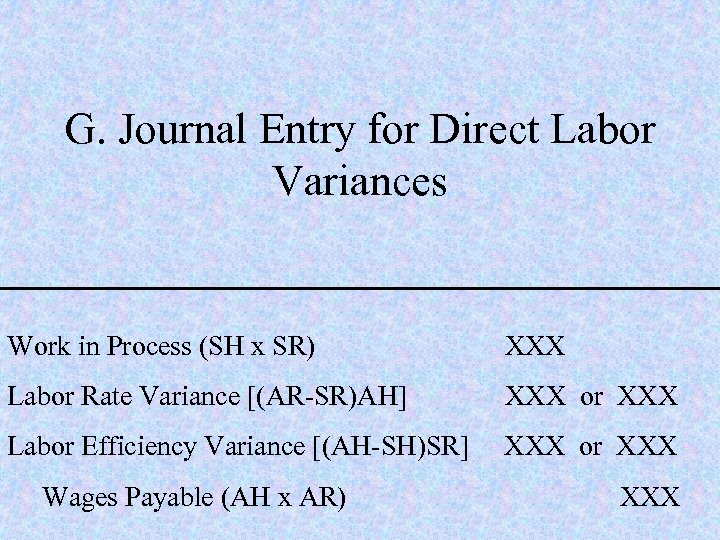G. Journal Entry for Direct Labor Variances Work in Process (SH x SR) XXX Labor Rate Variance [(AR-SR)AH] XXX or XXX Labor Efficiency Variance [(AH-SH)SR] XXX or XXX Wages Payable (AH x AR) XXX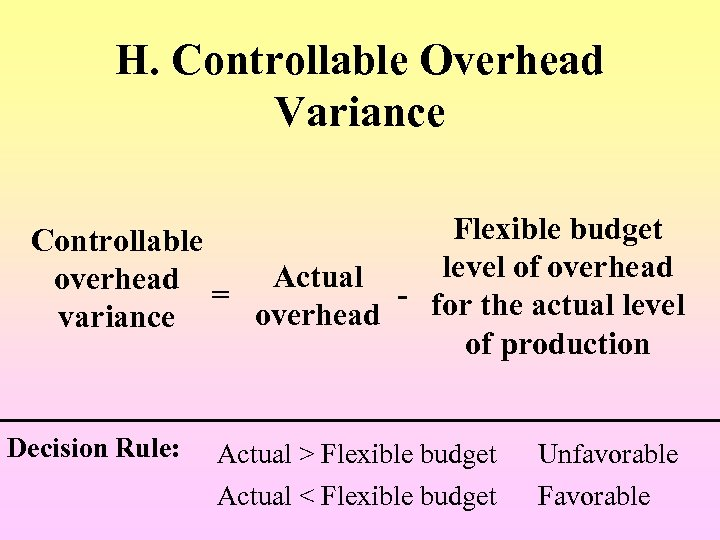H. Controllable Overhead Variance Flexible budget Controllable overhead = Actual - level of overhead for the actual level overhead variance of production Decision Rule: Actual > Flexible budget Actual < Flexible budget Unfavorable Favorable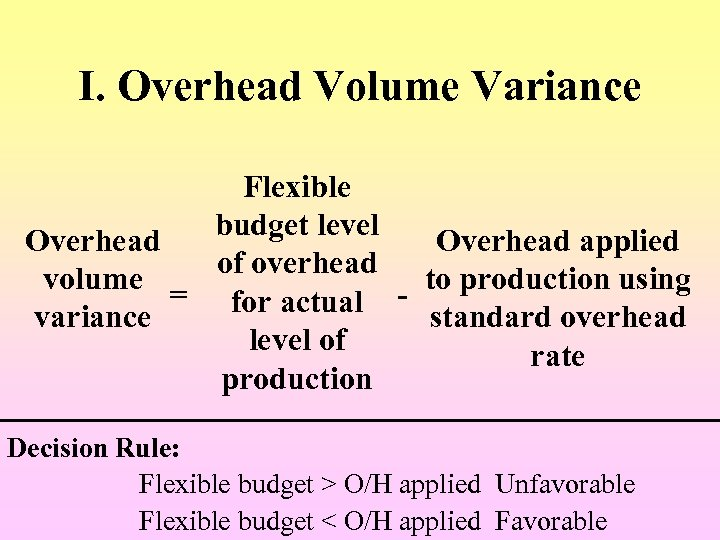I. Overhead Volume Variance Flexible budget level Overhead applied of overhead to production using volume = for actual - standard overhead variance level of rate production Decision Rule: Flexible budget > O/H applied Unfavorable Flexible budget < O/H applied Favorable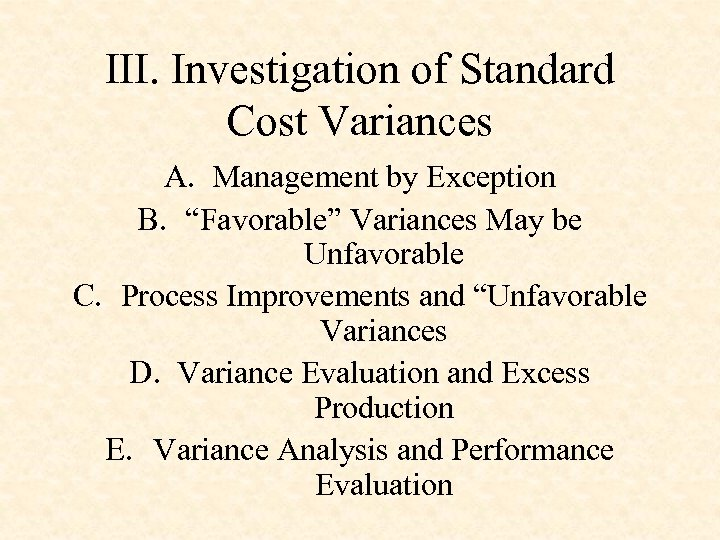III. Investigation of Standard Cost Variances A. Management by Exception B. “Favorable” Variances May be Unfavorable C. Process Improvements and “Unfavorable Variances D. Variance Evaluation and Excess Production E. Variance Analysis and Performance Evaluation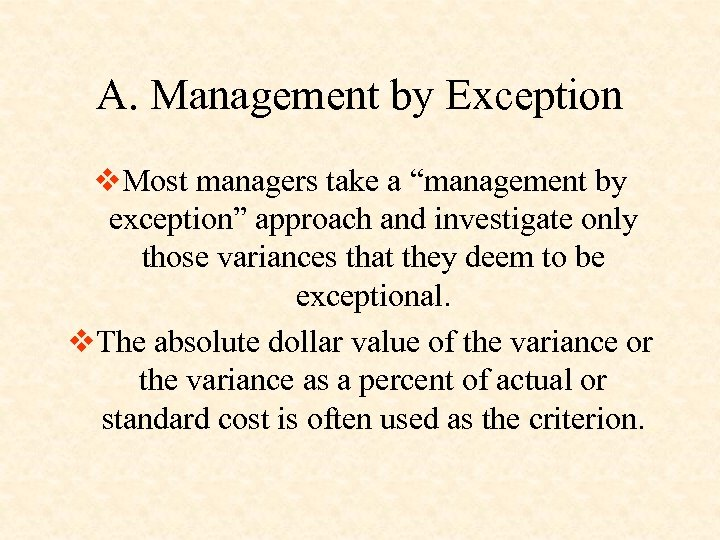A. Management by Exception v. Most managers take a “management by exception” approach and investigate only those variances that they deem to be exceptional. v. The absolute dollar value of the variance or the variance as a percent of actual or standard cost is often used as the criterion.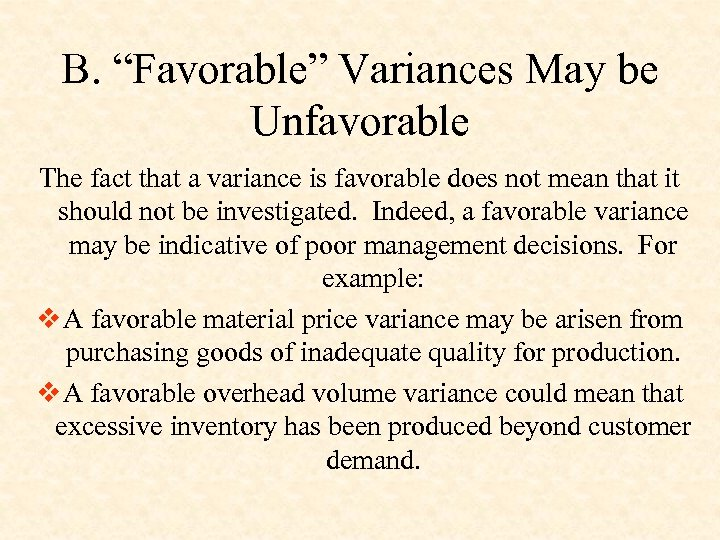B. “Favorable” Variances May be Unfavorable The fact that a variance is favorable does not mean that it should not be investigated. Indeed, a favorable variance may be indicative of poor management decisions. For example: v A favorable material price variance may be arisen from purchasing goods of inadequate quality for production. v A favorable overhead volume variance could mean that excessive inventory has been produced beyond customer demand.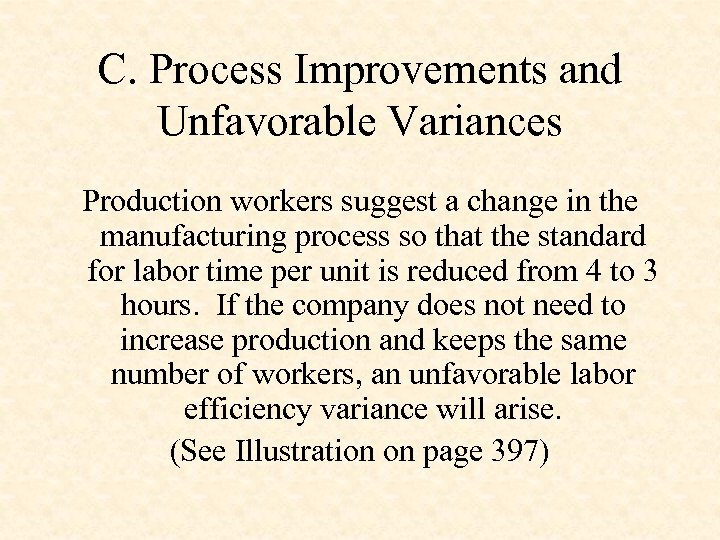C. Process Improvements and Unfavorable Variances Production workers suggest a change in the manufacturing process so that the standard for labor time per unit is reduced from 4 to 3 hours. If the company does not need to increase production and keeps the same number of workers, an unfavorable labor efficiency variance will arise. (See Illustration on page 397)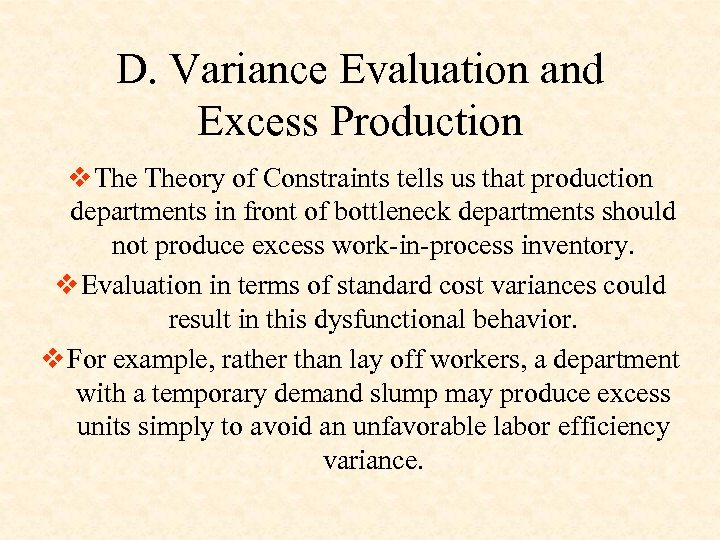D. Variance Evaluation and Excess Production v Theory of Constraints tells us that production departments in front of bottleneck departments should not produce excess work-in-process inventory. v Evaluation in terms of standard cost variances could result in this dysfunctional behavior. v For example, rather than lay off workers, a department with a temporary demand slump may produce excess units simply to avoid an unfavorable labor efficiency variance.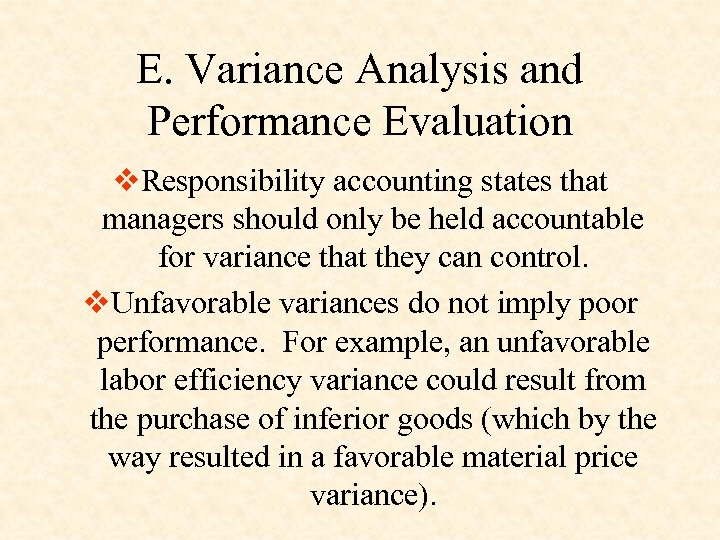E. Variance Analysis and Performance Evaluation v. Responsibility accounting states that managers should only be held accountable for variance that they can control. v. Unfavorable variances do not imply poor performance. For example, an unfavorable labor efficiency variance could result from the purchase of inferior goods (which by the way resulted in a favorable material price variance).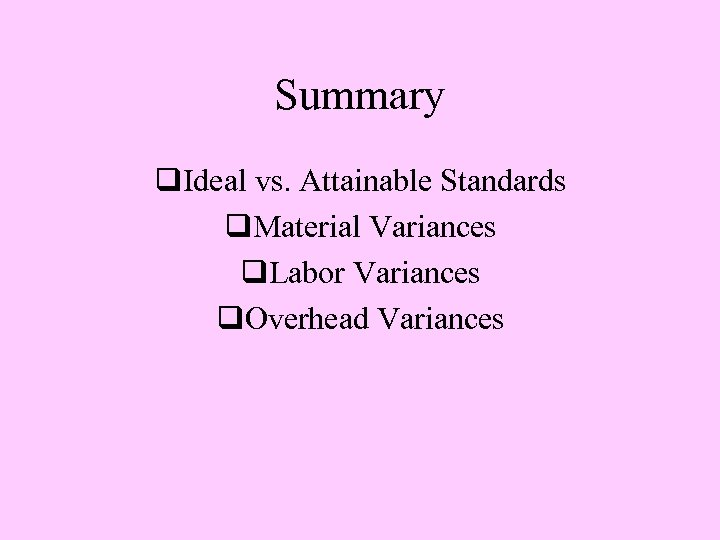Summary q. Ideal vs. Attainable Standards q. Material Variances q. Labor Variances q. Overhead Variances